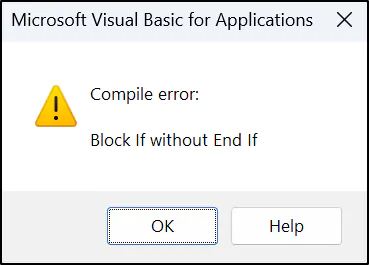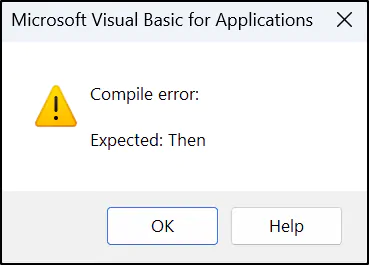# Understanding Excel VBA If Then Else StatementsAlan Murray#### Join the Excel conversation on Slack

Ask a question or join the conversation for all things Excel on our Slack channel.

The If Then Else statement is the most commonly used conditional construct in Excel VBA. You will find it in many procedures directing the flow of code by testing values and executing different statements dependent upon the result of the tests.

In this article, we will explain the syntax of the If Then Else statement, the different ways that it can be written, and as always, multiple examples of its use.

## Why use the 'If Then Else' statement?

The If Then Else statement is used to test a value and perform a statement or block of statements dependent upon the result of the test.

If Then Else can be used to perform a simple conditional test or extended to perform multiple conditional tests on a value. The And and Or keywords can also be used to combine conditions.

We will see a few different VBA code examples in this tutorial that demonstrate these different uses of the Excel VBA If Then Else statement.

## The syntax

The syntax of the If Then Else statement is quite easy to remember as it follows the words used in its name. It is also very similar to the structure of the IF function in Excel, which you have probably used before.

However, there are a few different ways to write the Excel VBA If Then Else statement and it can take some practice to get familiar with them.

### Option 1

When using the If statement to execute only one statement as a result of a condition, it can be written in single-line form, as follows.

If condition Then statement [Else statement]

The condition is any expression that evaluates to True or False. The required statement is then executed dependent on the result of the condition. The Else statement is optional and can therefore be omitted.

### Option 2

When executing a group of statements as a result of the condition, the If Then Else statement can be written in its block form syntax, as follows.

``````If condition Then
statements

Else
statements
End If``````

Notice the inclusion of the End If keywords on the last line. This was not required when writing If Then Else in its single-line syntax.

### Option 3

It is possible to nest If statements in Excel VBA by using the keyword ElseIf (written as one word). You can nest as many If statements as required.

The structure of the If statements is the same; remember to use the keyword Then at the end of the condition line.

``````If condition Then

statements

ElseIf condition n Then

statements
Else

statements

End If``````

## Missing keyword errors

At some time when writing your VBA code, you are sure to miss a word or get the different syntaxes of the If Then Else statement mixed up.

For example, you may type End If at the end of the single-line form when it is not required. Or, you may accidentally put a space between the words Else and If when using the ElseIf keyword.

The good news is that these mistakes are quite easy to recognize from the errors that are returned in the Visual Basic Editor. For example, the following error is shown when writing If Then Else in its block form and omitting the End If keywords at the end.And the following error is returned when omitting the keyword Then.You can see that these were quite identifiable by the helpful error description. Not all errors are so easy to identify, and it is useful to learn more about debugging VBA code (link to my debugging VBA code article here that is not yet published).

Now that we understand the syntax, let’s dive into some Excel VBA If Then Else statement examples to see it in action.

## Simple 'If Then Else' statement

In the following example, a single statement was executed for both the Then and Else scenarios. This is perfect for demonstrating the If Then Else statement in its single-line form.

The condition of the score being greater than or equal to 80 is tested, and “Pass” is assigned to the Result variable if the condition evaluates to True; otherwise “Fail” is assigned to the Result variable.

``````Sub ExamResults()

Dim Score As Byte, Result As String

Score = 81

If Score >= 80 Then Result = "Pass" Else Result = "Fail"

End Sub``````

## Omitting the 'Else' keyword

In the following example, a VBA procedure is used to protect only the cells that contain formulas on all sheets of a workbook.

An object variable has been declared for the worksheet named ws and this has been used in a For Each Next construct to loop through all sheets in the Worksheets collection.

The If Then Else statement has been added to check if the worksheet is protected, and if this is True, to unprotect the worksheet before continuing with the next statements in the procedure.

``````Sub ProtectSheets()

Dim ws As Worksheet

For Each ws In ActiveWorkbook.Worksheets

If ws.ProtectContents = True Then ws.Unprotect
.Cells.Locked = False
.Cells.SpecialCells(xlCellTypeFormulas).Locked = True
ws.Protect

Next ws

End Sub``````

This small Excel VBA If statement code example demonstrates the Else keyword being omitted as there was no alternative action to take in this case.

The single-line version of the If statement was used again as only one statement (to unprotect the worksheet) was executed as a result of the condition.

If ws.ProtectContents = True Then ws.Unprotect

## Excel VBA 'If Then Else' multiple statements

When executing multiple statements, the block form syntax of the If Then Else statement is used.

In this example, the Else statements are omitted again as there is no alternative action for if the condition evaluates to False.

``````Sub IfExample()

If Range("A2").Value = "" Then

MsgBox "The name has been omitted on the sheet."
Range("A2").Select
Exit Sub

End If

End Sub``````

This procedure is used to test the value in cell A2 of the active sheet to check if it is empty. If so, multiple statements are executed.

A message box is displayed informing the user that a name has not been entered into cell A2. Then cell A2 is selected so that it is active when the user is returned to the sheet. And finally, the Exit Sub keywords are used to exit the procedure.

## Nested 'If Then Else' statements

To perform multiple conditional tests on a value, you can nest If Then Else statements using the ElseIf keyword.

In the following example, the ExamResults procedure from a previous example is extended to assign four different results dependent upon the exam score attained.

``````Sub ExamResults()

Dim Score As Byte, Result As String

Score = 81

If Score >= 95 Then

Result = "Superb"

ElseIf Score >= 85 Then

Result = "Great"

ElseIf Score >= 75 Then

Result = "Good"

Else

Result = "Poor"

End If

End Sub``````

In this example, when testing numeric values in this manner, it is important that the conditional test against the largest value is performed first.

Otherwise, if performed in reverse, a score of 97 would return “Good” as it is greater than or equal to the value of 75 and there would be no need to test against the other conditions.

## Excel VBA 'If' statement with multiple conditions

For the final example, we will see the And and Or keywords used to extend the conditional tests that an If Then Else statement can perform.

The If Then Else statement in Excel VBA can handle a single condition only in isolation. But, the And and Or functions can be included to extend a simple VBA IF statement to multiple conditions.

They are simple to use, as you just need to use the keyword And or Or between each condition.

With the And keyword, both conditions on either side of And need to evaluate to True for the result to be True. And with Or, if either condition evaluates to True, then the result is True.

The following example is an extension of a previous code example. The IfExample procedure is testing if the values in ranges A2 or B2 are empty. If either of these tests evaluate to True, multiple statements are executed with the final statement exiting the procedure.

``````Sub IfExample()

If Range("A2").Value = "" Or Range("B2").Value = "" Then

MsgBox "One of the required values is missing."

Range("A2").Select

Exit Sub

End If

End Sub``````

## Key Takeaways

The If Then Else statement in Excel VBA is a commonly used conditional construct that is used to test a value and perform a statement or block of statements dependent upon the result of the test. It is important to understand the syntax of the statement and how to use it to perform simple or multiple conditional tests.

The And and Or keywords can be used to combine conditions, and it is possible to nest If statements. Common errors that can occur while writing If Then Else statements should be recognized and addressed. To become proficient in creating powerful macros in Excel, taking an online course with practical tutorials is recommended.

If you are looking to enhance your skills in Excel and VBA, GoSkills offers a wide range of online courses and resources that are designed to help individuals become proficient in Excel. These courses are taught by industry experts and cover everything from the basics of Excel to advanced features of VBA.

Become a certified Excel ninja with GoSkills bite-sized courses#### Join the Excel conversation on Slack

Ask a question or join the conversation for all things Excel on our Slack channel.Alan Murray

Alan is a Microsoft Excel MVP, Excel trainer and consultant. Most days he can be found in a classroom spreading his love and knowledge of Excel. When not in a classroom he is writing and teaching online through blogs, YouTube and podcasts. Alan lives in the UK, is a father of two and a keen runner.